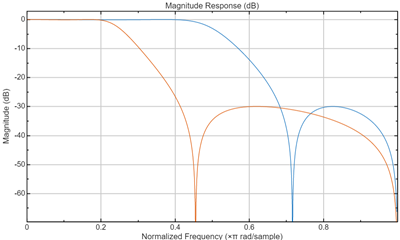# zpkftransf

Zero-pole-gain frequency transformation

## Syntax

```[Z2,P2,K2] = zpkftransf(Z,P,K,AllpassNum,AllpassDen) ```

## Description

`[Z2,P2,K2] = zpkftransf(Z,P,K,AllpassNum,AllpassDen)` returns zeros, `Z`2, poles, `P`2, and gain factor, `K`2, of the transformed lowpass digital filter. The prototype lowpass filter is given with zeros, `Z`, poles, `P`, and gain factor, `K`. If `AllpassDen` is not specified it will default to 1. If neither `AllpassNum` nor `AllpassDen` is specified, then the function returns the input filter.

## Examples

Design a prototype real IIR halfband filter using a standard elliptic approach:

```[b, a] = ellip(3,0.1,30,0.409); [AlpNum, AlpDen] = allpasslp2lp(0.5, 0.25); [z2, p2, k2] = zpkftransf(roots(b),roots(a),b(1),AlpNum,AlpDen);```

Verify the result by comparing the prototype filter with the target filter:

`fvtool(b, a, k2*poly(z2), poly(p2));`

After transforming the filter, you get the response shown in the figure, where the passband has been shifted towards zero.## Arguments

VariableDescription
`Z`

Zeros of the prototype lowpass filter

`P`

Poles of the prototype lowpass filter

`K`

Gain factor of the prototype lowpass filter

`FTFNum`

Numerator of the mapping filter

`FTFDen`

Denominator of the mapping filter

`Z2`

Zeros of the target filter

`P2`

Poles of the target filter

`K2`

Gain factor of the target filter